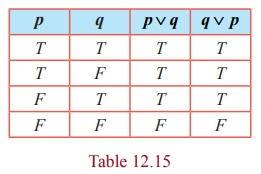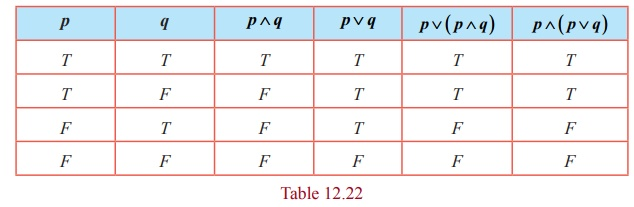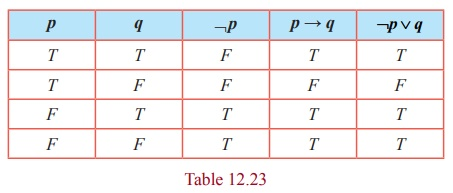Home | | Maths 12th Std | Some Laws of Logical Equivalence

# Some Laws of Logical Equivalence

Any two compound statements A and B are said to be logically equivalent or simply equivalent if the columns corresponding to A and B in the truth table have identical truth values.

Mathematical Logic

Logical Equivalence

### Definition 12.20

Any two compound statements A and B are said to be logically equivalent or simply equivalent if the columns corresponding to A and B in the truth table have identical truth values. The logical equivalence of the statements A and B is denoted by A B or A B .

From the definition, it is clear that, if A and B are logically equivalent, then A B must be tautology.

Some Laws of Equivalence

### 1. Idempotent Laws

(i) p p p

(ii) p p p .

ProofIn the above truth table for both p , p p and p p have the same truth values. Hence p p p and p p p .

### 2. Commutative Laws

(i) p q q p

(ii) p q q p .

Proof (i)The columns corresponding to p q and q p are identical. Hence p q q p .

Similarly (ii) p q q p can be proved.

### 3. Associative Laws

(i) p ( q r ) ( p q ) r (ii) p ( q r ) ( p q ) r .

### Proof

The truth table required for proving the associative law is given below.The columns corresponding to ( p q ) r and p ( q r ) are identical.

Hence p ( q r ) ( p q ) r .

Similarly, (ii) p ( q r ) ( p q ) r can be proved.

### 4. Distributive Laws

(i) p ( q r ) ( p q ) ( p r)

(ii) p ( q r ) ( p q ) ( p r)

Proof (i)The columns corresponding to p ( q r) and ( p q ) ( p r) are identical. Hence p ( q r ) ( p q ) ( p r) .

Similarly (ii) p ( q r ) ( p q ) ( p r) can be proved.

### 5. Identity Laws

(i) p T T and p F p

(ii) p T p and p FF(i) The entries in the columns corresponding to p T and T are identical and hence they are equivalent. The entries in the columns corresponding to p F and p are identical and hence they are equivalent.

Dually

(ii) p Tp and p FF can be proved.

### 6. Complement Laws

(i) p ¬ p T and p ¬ p F (ii) ¬T F and ¬F T

### Proof(i) The entries in the columns corresponding to p ¬ p and T are identical and hence they are equivalent. The entries in the columns corresponding to p ¬ p and F are identical and hence they are equivalent.

(ii) The entries in the columns corresponding to ¬T and F are identical and hence they are equivalent. The entries in the columns corresponding to ¬F and T are identical and hence they are equivalent.

¬(¬ p)p

### ProofThe entries in the columns corresponding to ¬ ( ¬p) and p are identical and hence they are equivalent.

### 8. de Morgan’s Laws

(i) ¬ ( p q) ¬ p ¬q

(ii) ¬ ( p q) ≡ ¬p ¬q

Proof of (i)The entries in the columns corresponding to ¬ ( p q ) and ¬ p ¬q are identical and hence they are equivalent. Therefore ¬ ( p q) ¬ p ¬q . Dually (ii) ¬ ( p q) ≡ ¬p ¬q can be proved.

### 9. Absorption Laws

(i) p ( p q ) p

(ii) p ( p q ) p(i) The entries in the columns corresponding to p ( p q) and p are identical and hence they are equivalent.

(ii) The entries in the columns corresponding to p ( p q) and p are identical and hence they are equivalent.

Example 12.17

Establish the equivalence property: p q ≡ ¬ p q

SolutionThe entries in the columns corresponding to p q and ¬ p q are identical and hence they are equivalent.

### Example 12.18

Establish the equivalence property connecting the bi-conditional with conditional:

pq( pq ) (qp)

### SolutionThe entries in the columns corresponding to p q and ( p → q ) ( q → p) are identical and hence they are equivalent.

### Example 12.19

Using the equivalence property, show that p q ( p q ) ( ¬ p ¬q) .

### Solution

It can be obtained by using examples 12.15 and 12.16 that

p q ( ¬ p q) ( ¬q p)

... (1)

(¬ p q) ( p ¬q) (by Commutative Law)

... (2)

( ¬ p ( p ¬q )) ( q ( p ¬q)) (by Distributive Law)

( ¬ p p) ( ¬p ¬q) ( q p) ( q ¬q) (by Distributive Law)

F ( ¬p ¬q) ( q p) F; (by Complement Law)

( ¬ p ¬ q ) ( q p) ; (by Identity Law)

( p q ) (¬ p ¬q) ; (by Commutative Law)

Finally (1) becomes p q ( p q ) (¬ p ¬q) .

Tags : Mathematical Logic | Discrete Mathematics | Mathematics , 12th Maths : UNIT 12 : Discrete Mathematics
Study Material, Lecturing Notes, Assignment, Reference, Wiki description explanation, brief detail
12th Maths : UNIT 12 : Discrete Mathematics : Some Laws of Logical Equivalence | Mathematical Logic | Discrete Mathematics | Mathematics